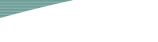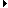HomeNews & ActivitiesDegrees OfferedCoursesAcademic StaffAdministrative StaffAdvisorsContact HourLaboratories and FacilitiesRelated LinksFaculty of Science and Technology

Seminar
Recurrence coefficients for discrete orthogonal polynomials with hypergeometric weight and discrete Painlevé equations
Speaker: Prof. Anton DZHAMAYProfessorSchool of Mathematical SciencesCollege of Natural and Health SciencesUniversity of Northern ColoradoU.S.A. 25 Oct 2019 (Friday) 12:00 - 13:00 E11-1009 Department of Mathematics

Abstract

Over the last decade it became clear that the role of discrete Painlevé equations in applications has been steadily growing. Thus, the question of recognizing a certain non-autonomous recurrence as a discrete Painlevé equation and understanding its position in Sakai’s classification scheme, recognizing whether it is equivalent to some known (model) example, and especially finding an explicit change of coordinates transforming it to such example, becomes one of the central ones. Fortunately, Sakai’s geometric theory provides an almost algorithmic procedure of answering this question. In this work we illustrate this procedure by studying an example coming from the theory of discrete orthogonal polynomials. There are many connections between orthogonal polynomials and Painlevé equations, both differential and discrete. In particular, often the coefficients of three-term recurrence relations for orthogonal polynomials can be expressed in terms of solutions of some discrete Painlevé equation. In this work we study orthogonal polynomials with general hypergeometric weight and show that their recurrence coefficients satisfy, after some change of variables, the standard discrete Painlevé-V equation. We also provide an explicit change of variables transforming this equation to the standard form. This is joint work with Galina Filipuk (University of Warsaw, Poland) and Alexander Stokes (University College, London, UK)

Biography

Prof. Anton Dzhamay got his undergraduate degree from Moscow Institute of Electronics and Mathematics and his PhD degree from Columbia University under the direction of Professor Igor Krichever. After a postdoc position at the University of Michigan in Ann Arbor, where he worked with Professor Igor Dolgachev, Anton moved to the University of Northern Colorado in 2005, where he is currently a Full Professor. Anton is interested in applications of algebro-geometric techniques to the study of various problems related to the theory of integrable systems and soliton equations, such as theory of Frobenius manifolds and WDVV equations, Whitham hierarchies, isospectral and isomonodromic transformations, and so on. Over the last 10 years his research has been on the geometric theory of discrete Painlevé equations (Sakai’s Theory) and its various applications.